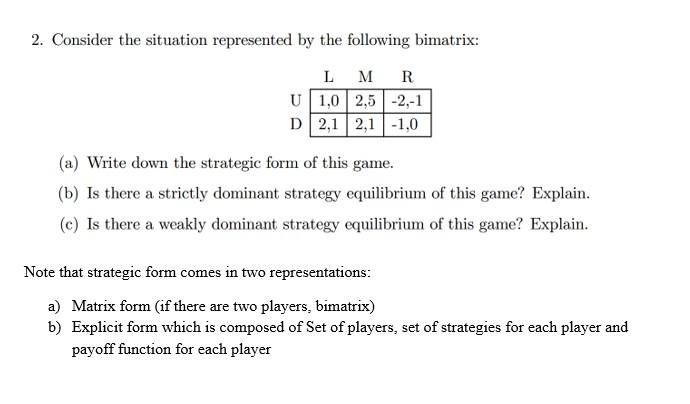# Question 2. Consider the situation represented by the following bimatrix: L M R U 1,0 2,5 -2,-1 D2,1 2,1 -1,0 (a) Write down the strategic form of this game. (b) Is there a strictly dominant strategy equilibrium of this game? Explain. (e) Is there a weakly dominant strategy equilibrium of this game? Explain. Note that strategic form comes in two representations: a) Matrix form (if there are two players, bimatrix) b) Explicit form which is composed of Set of players, set of strategies for each player and payoff function for each playerIYBRHT The Asker · Computer ScienceTranscribed Image Text: 2. Consider the situation represented by the following bimatrix: L M R U 1,0 2,5 -2,-1 D2,1 2,1 -1,0 (a) Write down the strategic form of this game. (b) Is there a strictly dominant strategy equilibrium of this game? Explain. (e) Is there a weakly dominant strategy equilibrium of this game? Explain. Note that strategic form comes in two representations: a) Matrix form (if there are two players, bimatrix) b) Explicit form which is composed of Set of players, set of strategies for each player and payoff function for each player
More
Transcribed Image Text: 2. Consider the situation represented by the following bimatrix: L M R U 1,0 2,5 -2,-1 D2,1 2,1 -1,0 (a) Write down the strategic form of this game. (b) Is there a strictly dominant strategy equilibrium of this game? Explain. (e) Is there a weakly dominant strategy equilibrium of this game? Explain. Note that strategic form comes in two representations: a) Matrix form (if there are two players, bimatrix) b) Explicit form which is composed of Set of players, set of strategies for each player and payoff function for each player